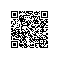# 数学专题1

NOIP2017 D1T1 小凯的疑惑

a*b-a-b

AK*1，MARK+=100；

NOIP2016 D2T1 组合数问题

NOIP2014 D2T3最后一道

~~~~~~~~~~~~~~~~~~~~

~~~~~~~~~~~~~~~~~~~~

~~~~~

质数

~~~~~

——————

质数判定

——————

15。

15/2=7······1,

15/3=5

SO

15不是质数。

77.

77/2=38······1

77/3=25······2

······

77/7=11

SO

77也不是质数。

13.

13/2=6······1

13/3=4······1

13/4=3······1

ceil（sqrt（13））（根号13取上整）=4，

code：

 1 bool prime(int x)
2 {
3       if(x<=1)   return 0;
4       if(x==2)   return 1;
5       for(int i=2;i<=sqrt(x);i++)
6       {
7             if(x%i==0)
8             return 0;
9       }
10       return 1;
11 }

code：

 1 memset(check,0,sizeof(check));//标记
2 int tot = 0;//质数个数
3 for(int i=2;i<=n;i++)
4 {
5     if(!check[i])//i没有别删去，则他是质数
6     {
7         prime[++tot]=i;
8     }
9     for(int j=1;j<=tot;j++)//去除i的倍数
10     {
11         if(i*prime[j]>n)//超过n，退出
12         {
13             break;
14         }
15         check[i*prime[j]]=1;//划去合数
16         if(i%prime[j]==0)
17         {
18             break;//合数被最小因子划去
19         }
20     }
21 } 

1、任何一个合数都可以表示成一个质数和一个数的成绩
2、假设A是一个合数，且A=x*y，这里x也是一个合数，那么有：
A=x*y（假设x是合数，y是质数）
x=a*b（假设a是质数，则a<x --> a<y)
A=a*b*y=a*Z (Z=b*y)
3、即一个合数x与一个质数y的乘积能表示成一个更大的合数Z和一个更小的质数a乘积表示

~~~~~~~~~~~~~

欧拉函数

~~~~~~~~~~~~~

code：

 1 int euler(int n){
2     int ans = n;
3     for(int i = 2; i * i <= n; i ++){
4         if(n % i == 0){
5             n /= i;
6             ans   = ans / i * (i-1);
7             while(n % i == 0){
8                 n /= i;
9             }
10         }
11     }
12     if(n > 1) ans = ans / n * (n - 1);
13     return ans;
14 }

φ（n） = n*(1-1/p1)*(1-1/p2)...使用钉钉扫一扫加入圈子
+ 订阅